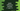# Difference between double and triple equal in Kotlin## Difference between == and === in Kotlin :

Double equal ”==” and triple equal ”===” are used for equality check in Kotlin. Both are different and not exactly same as like Java. In this post, we will see how these equal check works.

### Double equals :

Double equals ”==” is used for structural equality check. That means it checks if two variables contains equal data. It is different than double equal of Java. In Java, double equals == is used to compare reference of variables but in Kotlin it checks only data.

!= is the negated counterpart. It checks if two variables contains different data or not.

### Example :

``````fun main() {
val firstStr = "Hello"
val secondStr = buildString { append("Hello") }
val thirdStr = "Hello"

println(firstStr == secondStr)
println(firstStr == thirdStr)
}``````

Here, firstStr and thirdStr points to the same string object. Data in all variables are equal. Since, double equals only checks for data, both print statements will return ‘true’.

### Triple equals :

Triple equals ’===’ is used for referential equality, i.e. it checks if both variables are pointing to the same object or not. This is same as == of Java. For example, let’s try it with the above example :

``````fun main() {
val firstStr = "Hello"
val secondStr = buildString { append("Hello") }
val thirdStr = "Hello"

println(firstStr === secondStr)
println(firstStr === thirdStr)
}``````

It will print :

``````false
true``````

firstStr and thirdStr points to the same string objects. But secondStr is a different object.

Its negated counterpart is !==.